x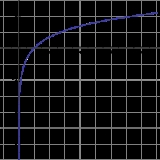Natural logarithmOverview

The natural logarithm is the logarithm
Logarithm
The logarithm of a number is the exponent by which another fixed value, the base, has to be raised to produce that number. For example, the logarithm of 1000 to base 10 is 3, because 1000 is 10 to the power 3: More generally, if x = by, then y is the logarithm of x to base b, and is written...

to the base e
E (mathematical constant)
The mathematical constant ' is the unique real number such that the value of the derivative of the function at the point is equal to 1. The function so defined is called the exponential function, and its inverse is the natural logarithm, or logarithm to base...

, where e is an irrational
Irrational number
In mathematics, an irrational number is any real number that cannot be expressed as a ratio a/b, where a and b are integers, with b non-zero, and is therefore not a rational number....

and transcendental
Transcendental number
In mathematics, a transcendental number is a number that is not algebraic—that is, it is not a root of a non-constant polynomial equation with rational coefficients. The most prominent examples of transcendental numbers are π and e...

constant approximately equal to 2.718281828. The natural logarithm is generally written as ln(x), loge(x) or sometimes, if the base of e is implicit, as simply log(x).

The natural logarithm of a number x is the power
Exponentiation
Exponentiation is a mathematical operation, written as an, involving two numbers, the base a and the exponent n...

to which e would have to be raised to equal x.Unanswered QuestionsChanging natural logarithm to real numberEncyclopedia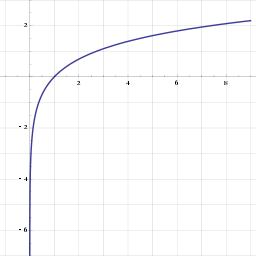The natural logarithm is the logarithm
Logarithm
The logarithm of a number is the exponent by which another fixed value, the base, has to be raised to produce that number. For example, the logarithm of 1000 to base 10 is 3, because 1000 is 10 to the power 3: More generally, if x = by, then y is the logarithm of x to base b, and is written...

to the base e
E (mathematical constant)
The mathematical constant ' is the unique real number such that the value of the derivative of the function at the point is equal to 1. The function so defined is called the exponential function, and its inverse is the natural logarithm, or logarithm to base...

, where e is an irrational
Irrational number
In mathematics, an irrational number is any real number that cannot be expressed as a ratio a/b, where a and b are integers, with b non-zero, and is therefore not a rational number....

and transcendental
Transcendental number
In mathematics, a transcendental number is a number that is not algebraic—that is, it is not a root of a non-constant polynomial equation with rational coefficients. The most prominent examples of transcendental numbers are π and e...

constant approximately equal to 2.718281828. The natural logarithm is generally written as ln(x), loge(x) or sometimes, if the base of e is implicit, as simply log(x).

The natural logarithm of a number x is the power
Exponentiation
Exponentiation is a mathematical operation, written as an, involving two numbers, the base a and the exponent n...

to which e would have to be raised to equal x. For example, ln(7.389...) is 2, because e2=7.389.... The natural log of e itself (ln(e)) is 1 because e1 = e, while the natural logarithm of 1 (ln(1)) is 0, since e0 = 1.

The natural logarithm can be defined for any positive real number
Real number
In mathematics, a real number is a value that represents a quantity along a continuum, such as -5 , 4/3 , 8.6 , √2 and π...

a as the area under the curve
Integral
Integration is an important concept in mathematics and, together with its inverse, differentiation, is one of the two main operations in calculus...

y = 1/x from 1 to a. The simplicity of this definition, which is matched in many other formulas involving the natural logarithm, leads to the term "natural." The definition can be extended to non-zero complex number
Complex number
A complex number is a number consisting of a real part and an imaginary part. Complex numbers extend the idea of the one-dimensional number line to the two-dimensional complex plane by using the number line for the real part and adding a vertical axis to plot the imaginary part...

s, as explained below.

The natural logarithm function, if considered as a real-valued function of a real variable, is the inverse function
Inverse function
In mathematics, an inverse function is a function that undoes another function: If an input x into the function ƒ produces an output y, then putting y into the inverse function g produces the output x, and vice versa. i.e., ƒ=y, and g=x...

of the exponential function
Exponential function
In mathematics, the exponential function is the function ex, where e is the number such that the function ex is its own derivative. The exponential function is used to model a relationship in which a constant change in the independent variable gives the same proportional change In mathematics,...

, leading to the identities: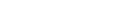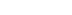Like all logarithms, the natural logarithm maps multiplication into addition: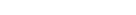Thus, the logarithm function is an isomorphism
Isomorphism
In abstract algebra, an isomorphism is a mapping between objects that shows a relationship between two properties or operations.  If there exists an isomorphism between two structures, the two structures are said to be isomorphic.  In a certain sense, isomorphic structures are...

from the group
Group (mathematics)
In mathematics, a group is an algebraic structure consisting of a set together with an operation that combines any two of its elements to form a third element. To qualify as a group, the set and the operation must satisfy a few conditions called group axioms, namely closure, associativity, identity...

of positive real numbers under multiplication to the group of real numbers under addition, represented as a function
Function (mathematics)
In mathematics, a function associates one quantity, the argument of the function, also known as the input, with another quantity, the value of the function, also known as the output. A function assigns exactly one output to each input. The argument and the value may be real numbers, but they can...

:Logarithms can be defined to any positive base other than 1, not just e; however logarithms in other bases differ only by a constant multiplier from the natural logarithm, and are usually defined in terms of the latter. Logarithms are useful for solving equations in which the unknown appears as the exponent of some other quantity. For example, logarithms are used to solve for the half-life, decay constant, or unknown time in exponential decay problems. They are important in many branches of mathematics and the sciences and are used in finance to solve problems involving compound interest
Compound interest
Compound interest arises when interest is added to the principal, so that from that moment on, the interest that has been added also itself earns interest. This addition of interest to the principal is called compounding...

.

## History

The first mention of the natural logarithm was by Nicholas Mercator
Nicholas Mercator
Nicholas Mercator , also known by his Germanic name Kauffmann, was a 17th-century mathematician....

in his work Logarithmotechnia published in 1668, although the mathematics teacher John Speidell had already in 1619 compiled a table on the natural logarithm. It was formerly also called hyperbolic logarithm, as it corresponds to the area under a hyperbola. It is also sometimes referred to as the Napierian logarithm
Napierian logarithm
The term Napierian logarithm, or Naperian logarithm, is often used to mean the natural logarithm. However, as first defined by John Napier, it is a function given by :...

, although the original meaning of this term is slightly different.

## Notational conventions

Mathematicians, statisticians, and some engineers generally understand either "log(x)" or "ln(x)" to mean loge(x), i.e., the natural logarithm of x, and write "log10(x)" if the base 10 logarithm
Common logarithm
The common logarithm is the logarithm with base 10. It is also known as the decadic logarithm, named after its base. It is indicated by log10, or sometimes Log with a capital L...

of x is intended.

Some engineers, biologists, and some others generally write "ln(x)" (or occasionally "loge(x)") when they mean the natural logarithm of x, and take "log(x)" to mean log10(x).

In most commonly-used programming language
Programming language
A programming language is an artificial language designed to communicate instructions to a machine, particularly a computer. Programming languages can be used to create programs that control the behavior of a machine and/or to express algorithms precisely....

s, including C
C (programming language)
C is a general-purpose computer programming language developed between 1969 and 1973 by Dennis Ritchie at the Bell Telephone Laboratories for use with the Unix operating system....

, C++
C++
C++ is a statically typed, free-form, multi-paradigm, compiled, general-purpose programming language. It is regarded as an intermediate-level language, as it comprises a combination of both high-level and low-level language features. It was developed by Bjarne Stroustrup starting in 1979 at Bell...

, SAS
SAS System
SAS is an integrated system of software products provided by SAS Institute Inc. that enables programmers to perform:* retrieval, management, and mining* report writing and graphics* statistical analysis...

, MATLAB
MATLAB
MATLAB is a numerical computing environment and fourth-generation programming language. Developed by MathWorks, MATLAB allows matrix manipulations, plotting of functions and data, implementation of algorithms, creation of user interfaces, and interfacing with programs written in other languages,...

, Fortran
Fortran
Fortran is a general-purpose, procedural, imperative programming language that is especially suited to numeric computation and scientific computing...

, and BASIC, "log" or "LOG" refers to the natural logarithm.

In hand-held calculator
Calculator
An electronic calculator is a small, portable, usually inexpensive electronic device used to perform the basic operations of arithmetic. Modern calculators are more portable than most computers, though most PDAs are comparable in size to handheld calculators.The first solid-state electronic...

s, the natural logarithm is denoted ln, whereas log is the base 10 logarithm.

In theoretical computer science
Theoretical computer science
Theoretical computer science is a division or subset of general computer science and mathematics which focuses on more abstract or mathematical aspects of computing....

, information theory, and cryptography "log(x)" generally means "log2
Binary logarithm
In mathematics, the binary logarithm is the logarithm to the base 2. It is the inverse function of n ↦ 2n. The binary logarithm of n is the power to which the number 2 must be raised to obtain the value n. This makes the binary logarithm useful for anything involving powers of 2,...

(x)" (although this is often written as lg(x) instead).

## Origin of the term natural logarithm

Initially, it might seem that since our numbering system is base 10, this base would be more "natural" than base e. But mathematically, the number 10 is not particularly significant. Its use culturally—as the basis for many societies’ numbering systems—likely arises from humans’ typical number of fingers. Other cultures have based their counting systems on such choices as 5
Quinary
Quinary is a numeral system with five as the base. A possible origination of a quinary system is that there are five fingers on either hand. The base five is stated from 0-4...

, 8
Octal
The octal numeral system, or oct for short, is the base-8 number system, and uses the digits 0 to 7. Numerals can be made from binary numerals by grouping consecutive binary digits into groups of three...

, 12
Duodecimal
The duodecimal system is a positional notation numeral system using twelve as its base. In this system, the number ten may be written as 'A', 'T' or 'X', and the number eleven as 'B' or 'E'...

, 20
Vigesimal
The vigesimal or base 20 numeral system is based on twenty .- Places :...

, and 60.

loge is a "natural" log because it automatically springs from, and appears so often in, mathematics. For example, consider the problem of differentiating
Derivative
In calculus, a branch of mathematics, the derivative is a measure of how a function changes as its input changes. Loosely speaking, a derivative can be thought of as how much one quantity is changing in response to changes in some other quantity; for example, the derivative of the position of a...

a logarithmic function:If the base b equals e, then the derivative is simply 1/x, and at x = 1 this derivative equals 1. Another sense in which the base-e-logarithm is the most natural is that it can be defined quite easily in terms of a simple integral or Taylor series
Taylor series
In mathematics, a Taylor series is a representation of a function as an infinite sum of terms that are calculated from the values of the function's derivatives at a single point....

and this is not true of other logarithms.

Further senses of this naturalness make no use of calculus
Calculus
Calculus is a branch of mathematics focused on limits, functions, derivatives, integrals, and infinite series. This subject constitutes a major part of modern mathematics education. It has two major branches, differential calculus and integral calculus, which are related by the fundamental theorem...

. As an example, there are a number of simple series involving the natural logarithm. Pietro Mengoli
Pietro Mengoli
Pietro Mengoli was an Italian mathematician and clergyman from Bologna, where he studied with Bonaventura Cavalieri at the University of Bologna, and succeeded him in 1647...

and Nicholas Mercator
Nicholas Mercator
Nicholas Mercator , also known by his Germanic name Kauffmann, was a 17th-century mathematician....

called it logarithmus naturalis a few decades before Newton and Leibniz developed calculus.

## Definitions

Formally, ln(a) may be defined as the area under the graph of 1/x from 1 to a, that is as the integral
Integral
Integration is an important concept in mathematics and, together with its inverse, differentiation, is one of the two main operations in calculus...

,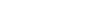This defines a logarithm because it satisfies the fundamental property of a logarithm:This can be demonstrated by letting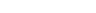as follows: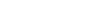The number e
E (mathematical constant)
The mathematical constant ' is the unique real number such that the value of the derivative of the function at the point is equal to 1. The function so defined is called the exponential function, and its inverse is the natural logarithm, or logarithm to base...

can then be defined as the unique real number a such that ln(a) = 1.

Alternatively, if the exponential function
Exponential function
In mathematics, the exponential function is the function ex, where e is the number such that the function ex is its own derivative. The exponential function is used to model a relationship in which a constant change in the independent variable gives the same proportional change In mathematics,...

has been defined first using an infinite series, the natural logarithm may be defined as its inverse function
Inverse function
In mathematics, an inverse function is a function that undoes another function: If an input x into the function ƒ produces an output y, then putting y into the inverse function g produces the output x, and vice versa. i.e., ƒ=y, and g=x...

, i.e., ln is that function such that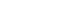. Since the range of the exponential function on real arguments is all positive real numbers and since the exponential function is strictly increasing, this is well-defined for all positive x.

## Properties

•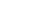•(see complex logarithm
Complex logarithm
In complex analysis, a complex logarithm function is an "inverse" of the complex exponential function, just as the natural logarithm ln x is the inverse of the real exponential function ex. Thus, a logarithm of z is a complex number w such that ew = z. The notation for such a w is log z...

)

•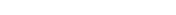•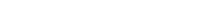•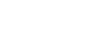## Derivative, Taylor series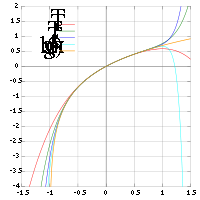The derivative
Derivative
In calculus, a branch of mathematics, the derivative is a measure of how a function changes as its input changes. Loosely speaking, a derivative can be thought of as how much one quantity is changing in response to changes in some other quantity; for example, the derivative of the position of a...

of the natural logarithm is given by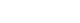This leads to the Taylor series
Taylor series
In mathematics, a Taylor series is a representation of a function as an infinite sum of terms that are calculated from the values of the function's derivatives at a single point....

foraround 0; also known as the Mercator series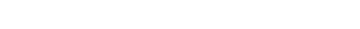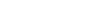At right is a picture of ln(1 + x) and some of its Taylor polynomials around 0. These approximations converge to the function only in the region −1 < x ≤ 1; outside of this region the higher-degree Taylor polynomials are worse approximations for the function.

Substituting x − 1 for x, we obtain an alternative form for ln(x) itself, namely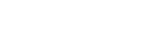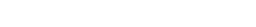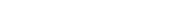By using the Euler transform on the Mercator series, one obtains the following, which is valid for any x with absolute value greater than 1: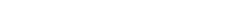This series is similar to a BBP-type formula.

Also note that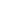is its own inverse function, so to yield the natural logarithm of a certain number y, simply put in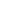for x.

## The natural logarithm in integration

The natural logarithm allows simple integration
Integral
Integration is an important concept in mathematics and, together with its inverse, differentiation, is one of the two main operations in calculus...

of functions of the form g(x) = f '(x)/f(x): an antiderivative
Antiderivative
In calculus, an "anti-derivative", antiderivative, primitive integral or indefinite integralof a function f is a function F whose derivative is equal to f, i.e., F ′ = f...

of g(x) is given by ln(|f(x)|). This is the case because of the chain rule
Chain rule
In calculus, the chain rule is a formula for computing the derivative of the composition of two or more functions. That is, if f is a function and g is a function, then the chain rule expresses the derivative of the composite function in terms of the derivatives of f and g.In integration, the...

and the following fact: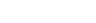In other words,and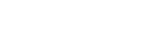Here is an example in the case of g(x) = tan(x):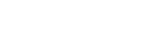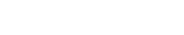Letting f(x) = cos(x) and f(x)= – sin(x):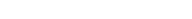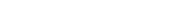where C is an arbitrary constant of integration
Arbitrary constant of integration
In calculus, the indefinite integral of a given function is only defined up to an additive constant, the constant of integration. This constant expresses an ambiguity inherent in the construction of antiderivatives...

.

The natural logarithm can be integrated using integration by parts
Integration by parts
In calculus, and more generally in mathematical analysis, integration by parts is a rule that transforms the integral of products of functions into other integrals...

:## Numerical value

To calculate the numerical value of the natural logarithm of a number, the Taylor series expansion can be rewritten as: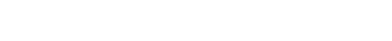To obtain a better rate of convergence, the following identity can be used.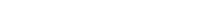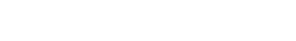provided that y = (x−1)/(x+1) and x > 0.

For ln(x) where x > 1, the closer the value of x is to 1, the faster the rate of convergence. The identities associated with the logarithm can be leveraged to exploit this: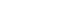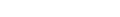Such techniques were used before calculators, by referring to numerical tables and performing manipulations such as those above.

### High precision

To compute the natural logarithm with many digits of precision, the Taylor series approach is not efficient since the convergence is slow. An alternative is to use Newton's method
Newton's method
In numerical analysis, Newton's method , named after Isaac Newton and Joseph Raphson, is a method for finding successively better approximations to the roots of a real-valued function. The algorithm is first in the class of Householder's methods, succeeded by Halley's method...

to invert the exponential function, whose series converges more quickly.

An alternative for extremely high precision calculation is the formula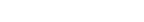where M denotes the arithmetic-geometric mean of 1 and 4/s, andwith m chosen so that p bits of precision is attained. (For most purposes, the value of 8 for m is sufficient.) In fact, if this method is used, Newton inversion of the natural logarithm may conversely be used to calculate the exponential function efficiently. (The constants ln 2 and π
Pi
' is a mathematical constant that is the ratio of any circle's circumference to its diameter. is approximately equal to 3.14. Many formulae in mathematics, science, and engineering involve , which makes it one of the most important mathematical constants...

can be pre-computed to the desired precision using any of several known quickly converging series.)

### Computational complexity

The computational complexity
Computational Complexity
Computational Complexity may refer to:*Computational complexity theory*Computational Complexity...

of computing the natural logarithm (using the arithmetic-geometric mean) is O(M(n) ln n). Here n is the number of digits of precision at which the natural logarithm is to be evaluated and M(n) is the computational complexity of multiplying two n-digit numbers.

## Continued fractions

While no simple continued fraction
Continued fraction
In mathematics, a continued fraction is an expression obtained through an iterative process of representing a number as the sum of its integer part and the reciprocal of another number, then writing this other number as the sum of its integer part and another reciprocal, and so on...

s are available, several generalized continued fraction
Generalized continued fraction
In complex analysis, a branch of mathematics, a generalized continued fraction is a generalization of regular continued fractions in canonical form, in which the partial numerators and partial denominators can assume arbitrary real or complex values....

s are, including: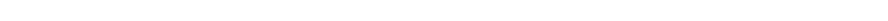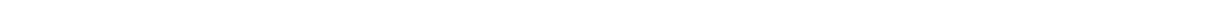## Complex logarithms

The exponential function can be extended to a function which gives a complex number
Complex number
A complex number is a number consisting of a real part and an imaginary part. Complex numbers extend the idea of the one-dimensional number line to the two-dimensional complex plane by using the number line for the real part and adding a vertical axis to plot the imaginary part...

as ex for any arbitrary complex number x; simply use the infinite series with x complex. This exponential function can be inverted to form a complex logarithm that exhibits most of the properties of the ordinary logarithm. There are two difficulties involved: no x has ex = 0; and it turns out that e2πi = 1 = e0. Since the multiplicative property still works for the complex exponential function, ez = ez+2nπi, for all complex z and integers n.

So the logarithm cannot be defined for the whole complex plane
Complex plane
In mathematics, the complex plane or z-plane is a geometric representation of the complex numbers established by the real axis and the orthogonal imaginary axis...

, and even then it is multi-valued – any complex logarithm can be changed into an "equivalent" logarithm by adding any integer multiple of 2πi at will. The complex logarithm can only be single-valued on the cut plane. For example, ln i = 1/2 πi or 5/2 πi or −3/2 πi, etc.; and although i4 = 1, 4 log i can be defined as 2πi, or 10πi or −6 πi, and so on.

## See also

• John Napier
John Napier
John Napier of Merchiston – also signed as Neper, Nepair – named Marvellous Merchiston, was a Scottish mathematician, physicist, astronomer & astrologer, and also the 8th Laird of Merchistoun. He was the son of Sir Archibald Napier of Merchiston. John Napier is most renowned as the discoverer...

– inventor of logarithms
• Logarithm of a matrix
Logarithm of a matrix
In mathematics, a logarithm of a matrix is another matrix such that the matrix exponential of the latter matrix equals the original matrix. It is thus a generalization of the scalar logarithm and in some sense an inverse function of the matrix exponential. Not all matrices have a logarithm and...

• Logarithmic integral function
Logarithmic integral function
In mathematics, the logarithmic integral function or integral logarithm li is a special function. It occurs in problems of physics and has number theoretic significance, occurring in the prime number theorem as an estimate of the number of prime numbers less than a given value.-Integral...

• Nicholas Mercator
Nicholas Mercator
Nicholas Mercator , also known by his Germanic name Kauffmann, was a 17th-century mathematician....

– first to use the term natural log
• Polylogarithm
• Von Mangoldt function
Von Mangoldt function
In mathematics, the von Mangoldt function is an arithmetic function named after German mathematician Hans von Mangoldt.-Definition:The von Mangoldt function, conventionally written as Λ, is defined as...

• The number e
E (mathematical constant)
The mathematical constant ' is the unique real number such that the value of the derivative of the function at the point is equal to 1. The function so defined is called the exponential function, and its inverse is the natural logarithm, or logarithm to base...

• Natural logarithm of 2
• Leonhard Euler
Leonhard Euler
Leonhard Euler was a pioneering Swiss mathematician and physicist. He made important discoveries in fields as diverse as infinitesimal calculus and graph theory. He also introduced much of the modern mathematical terminology and notation, particularly for mathematical analysis, such as the notion...

## External links

The source of this article is wikipedia, the free encyclopedia.  The text of this article is licensed under the GFDL.